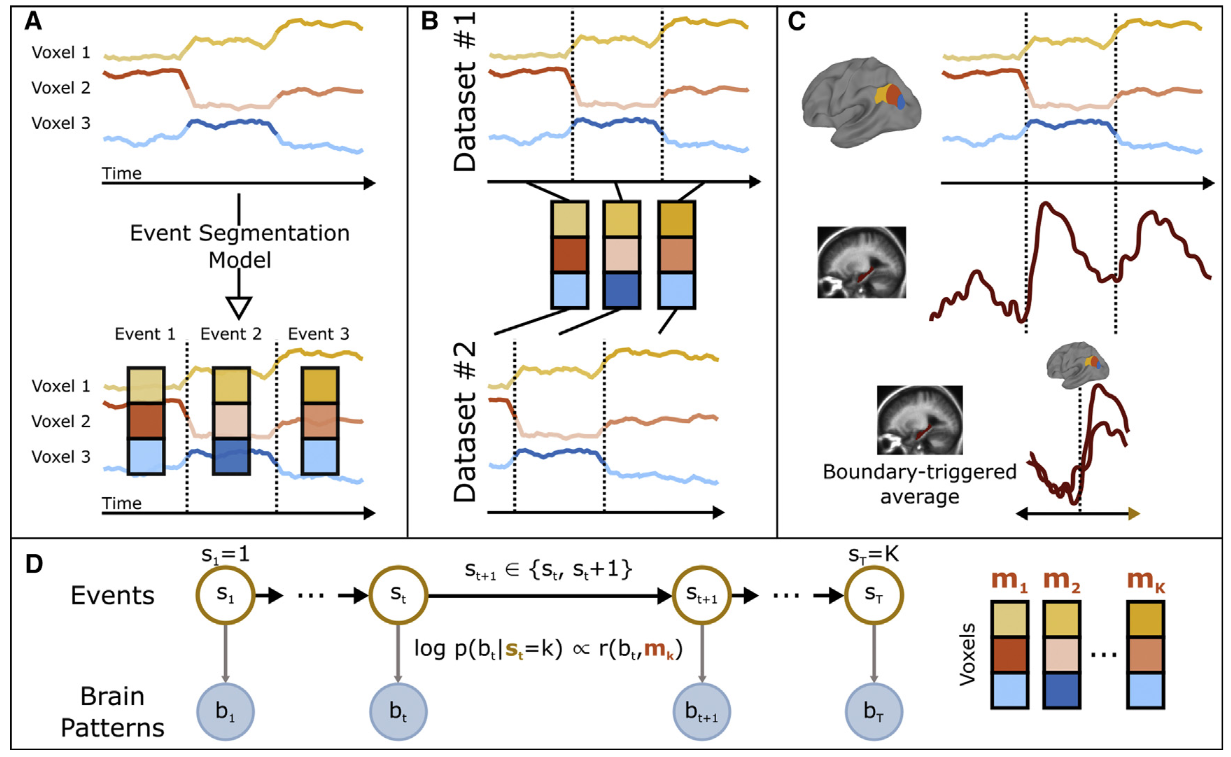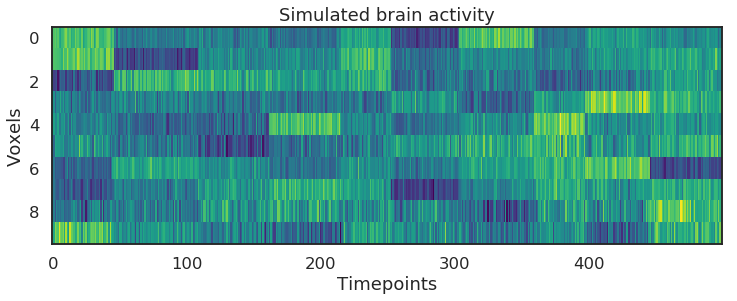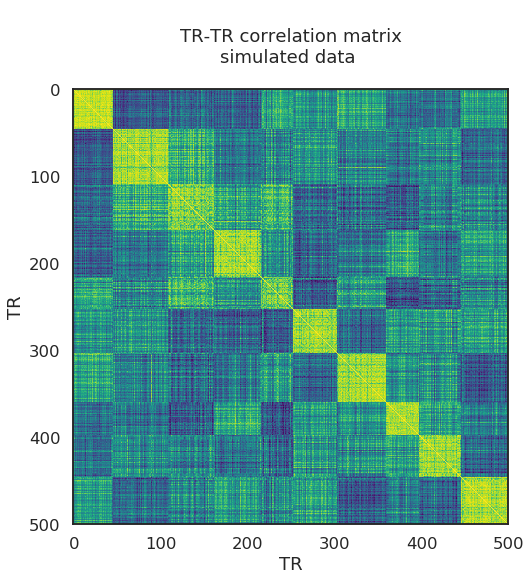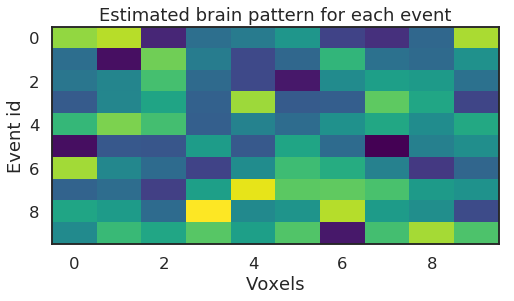# Hidden Markov Models for Event Segmentation¶

Contributions

If asked to give a quick description of a dinner with friends, you might say something like the following: "First, we met outside the restaurant while waiting for our table. Once we got to our table, we continued talking for a bit before we ordered something to drink and a few appetizers. Later, everyone ordered their dinner. The food arrived after some time and we all began eating. Finally, it was time for dessert and we continued chatting with each other until desert arrived. After this, we split the bill and headed out of the restaurant. We said our goodbyes to each other, while waiting for our taxis, and went home." From this description it is clear that the dinner meeting was composed of stages, or events, that occurred sequentially. Furthermore, these events can be perceived at varying scales. At the longest time scale, the entire dinner could be treated as one event. At smaller time scales, subsets of the meeting such as entering the restaurant, taking off coats, being seated, looking at menus and so on, can be treated as different events. At an even smaller scale, the event of entering the restaurant can be broken up into different sub-events. Regardless of scale, all of these accounts share the property that the event can be represented as a sequence of stages.

The goal of this notebook is to explore methods for finding these “sequence-of-stages” representations in the brain. To accomplish this, we use a machine learning technique called Hidden Markov Modeling (HMM). These models assume that your thoughts progress through a sequence of states, one at a time. You can’t directly observe people’s thoughts, so the states are “hidden” (not directly observable). However, you can directly observe BOLD activity. So the full specification of the HMM is that each “hidden” state (corresponding to thinking about a particular stage of an event) has an observable BOLD correlate (specifically, a spatial pattern across voxels). The goal of HMM analysis is to take the BOLD data timeseries and then infer the sequence of states that the participant’s brain went through, based on the BOLD activity. Note that the broadest formulation of an HMM allows all possible transitions between states (e.g., with three states, some probability of transitioning from S1 to S2, S1 to S3, S2 to S1, S2 to S3, S3 to S1, S3 to S2). However, in the formulation of the HMM used here, we will assume that the participant’s brain can only progress forward between adjacent states (S1 to S2, S2 to S3). This more limited formulation of the HMM is well-suited to situations where we know that events proceed in a stereotyped sequence (e.g., we know that the waiter brings the food after you order the food, not the other way around).

In summary: The HMM analysis used here assumes that the time series of BOLD activity was generated by the participant’s brain progressing through a sequence of states, where each state corresponds to a spatial pattern of BOLD activity. Intuitively, when we do HMM analysis, we are trying to identify when the brain has transitioned from one hidden state to another, by looking at the BOLD time series and estimating when the spatial pattern has switched. By fitting HMM with different numbers of hidden states, we can determine how many states a brain region traverses and identify when the transitions occur. Here we will apply the HMM to fMRI data from watching and recalling a movie (from Chen et al., 2017), identifying how different brain regions chunk the movie at different time scales, and examining where in the brain recalling a movie evokes the same sequence of neural patterns as viewing the movie. A full description is given in Baldassano et al. (2017).A schematic diagram of HMM, from Baldassano et al. (2017).

• (A) Given a set of (unlabeled) time courses from a region of interest, the goal of the event segmentation model is to temporally divide the data into ‘‘events’’ with stable activity patterns, punctuated by ‘‘event boundaries’’ at which activity patterns rapidly transition to a new stable pattern. The number and locations of these event boundaries can then be compared across brain regions or to stimulus annotations.
• (B) The model can identify event correspondences between datasets (e.g., responses to movie and audio versions of the same narrative) that share the same sequence of event activity patterns, even if the duration of the events is different.
• (C) The model-identified boundaries can also be used to study processing evoked by event transitions, such as changes in hippocampal activity coupled to event transitions in the cortex.
• (D) The event segmentation model is implemented as a modified Hidden Markov Model (HMM) in which the latent state $s_t$ for each time point denotes the event to which that time point belongs, starting in event 1 and ending in event $K$. All datapoints during event $k$ are assumed to be exhibit high similarity with an event-specific pattern $m_k$.

## Goal of this script¶

1. Understand how HMMs can be useful for analyzing time series.
2. Learn how to run HMM in BrainIAK.
3. Use HMM to infer events from brain activity.
4. Determine the statistical significance from the HMM.



2. Comparing model boundaries to human-labeled boundaries

3. Aligning movie and recall data

#### Exercises¶

In :
import warnings
import sys
import os
# import logging

import deepdish as dd
import numpy as np

import brainiak.eventseg.event
import nibabel as nib

import scipy.io
from scipy import stats
from scipy.stats import norm, zscore, pearsonr
from scipy.signal import gaussian, convolve
from sklearn import decomposition
from sklearn.model_selection import LeaveOneOut, KFold

from matplotlib import pyplot as plt
from mpl_toolkits.mplot3d import Axes3D
import matplotlib.patches as patches
import seaborn as sns

%autosave 5
%matplotlib inline
sns.set(style = 'white', context='talk', font_scale=1, rc={"lines.linewidth": 2})

if not sys.warnoptions:
warnings.simplefilter("ignore")

from utils import sherlock_h5_data

if not os.path.exists(sherlock_h5_data):
os.makedirs(sherlock_h5_data)
print('Make dir: ', sherlock_h5_data)
else:
print('Data path exists')

from utils import sherlock_dir

Autosaving every 5 seconds
Data path exists


## 0. HMM with toy data ¶

Here we introduce the usage of HMM in BrainIAK with a toy simulation. In particular, we will generate data with a known number $K$ of event boundaries. The following steps are used to run this simulation:

1. Create event labels for each time-point in the toy dataset using generate_event_labels.
2. Create data with these event labels using generate_data.
3. Check the data matrix to see if we have created events -- time periods where the signal is relatively constant.
4. Segment the signal using the brainiak HMM function: brainiak.eventseg.event.EventSegment(K).
5. Overlay the results from HMM with the ground truth segments from the data.
In :
def generate_event_labels(T, K, length_std):
event_labels = np.zeros(T, dtype=int)
start_TR = 0
for e in range(K - 1):
length = round(
((T - start_TR) / (K - e)) * (1 + length_std * np.random.randn()))
length = min(max(length, 1), T - start_TR - (K - e))
event_labels[start_TR:(start_TR + length)] = e
start_TR = start_TR + length
event_labels[start_TR:] = K - 1

return event_labels

def generate_data(V, T, event_labels, event_means, noise_std):
simul_data = np.empty((V, T))
for t in range(T):
simul_data[:, t] = stats.multivariate_normal.rvs(
event_means[:, event_labels[t]], cov=noise_std, size=1)

simul_data = stats.zscore(simul_data, axis=1, ddof=1)
return simul_data


Create and plot simulated data. Imagine the following matrix is the voxel by TR bold activity matrix, averaged across participants.

In :
# Parameters for creating small simulated datasets
V = 10 # number of voxels
K = 10 # number of events
T = 500 # Time points

# Generate the first dataset
np.random.seed(1)
event_means = np.random.randn(V, K)
event_labels = generate_event_labels(T, K, 0.2)
D = generate_data(V, T, event_labels, event_means, 1/4)

# Check the data.
f, ax = plt.subplots(1,1, figsize=(12, 4))
ax.imshow(D, interpolation='nearest', cmap='viridis', aspect='auto')
ax.set_ylabel('Voxels')
ax.set_title('Simulated brain activity')
ax.set_xlabel('Timepoints')

Out:
Text(0.5, 0, 'Timepoints')The goal of the HMM is to identify chunks of time during which activity patterns remain relatively constant. To see if this is a reasonable model for our dataset, we can plot a timepoint-timepoint correlation matrix, showing the similarity between every pair of timepoints in our dataset (averaged over subjects).

In :
f, ax = plt.subplots(1,1, figsize = (10,8))
ax.imshow(np.corrcoef(D.T), cmap='viridis')
title_text = '''
TR-TR correlation matrix
simulated data
'''
ax.set_title(title_text)
ax.set_xlabel('TR')
ax.set_ylabel('TR')

Out:
Text(0, 0.5, 'TR')Calling brainiak.eventseg.event.EventSegment(k) fits a hidden markov model with parameter k, where k is your guess about how many events (or brain states) are in the data. Here we are using the ground truth number of events.

In :
# Find the events in this dataset
hmm_sim = brainiak.eventseg.event.EventSegment(K)
hmm_sim.fit(D.T)

Out:
EventSegment(event_chains=array([0., 0., 0., 0., 0., 0., 0., 0., 0., 0.]),
n_events=10, n_iter=500,
step_var=<function EventSegment._default_var_schedule at 0x7fe52efec950>)

Another output of the event segmentation fit is the estimated activity pattern for each event: HMM.event_pat_.

In :
f, ax = plt.subplots(1,1, figsize=(8, 4))

ax.imshow(hmm_sim.event_pat_.T, cmap='viridis', aspect='auto')
ax.set_title('Estimated brain pattern for each event')
ax.set_ylabel('Event id')
ax.set_xlabel('Voxels')

Out:
Text(0.5, 0, 'Voxels')One way of visualizing the fit is to mark on the timepoint correlation matrix where the model thinks the borders of the events are. The (soft) event segmentation is in HMM.segments_, so we can convert this to hard bounds by taking the argmax.

In :
# plot
f, ax = plt.subplots(1,1, figsize=(12,4))

pred_seg = hmm_sim.segments_
ax.imshow(pred_seg.T, aspect='auto', cmap='viridis')
ax.set_xlabel('Timepoints')
ax.set_ylabel('Event label')
ax.set_title('Predicted event segmentation, by HMM with the ground truth n_events')

f.tight_layout()